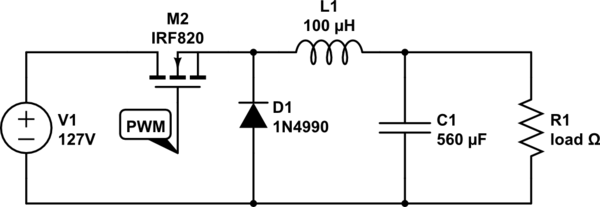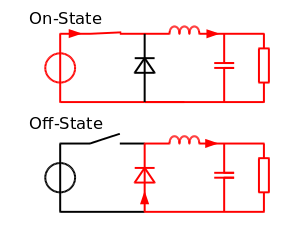# Buck converter simulation using PSpice : tutorial 8

In this tutorial I will explain you the working of a buck converter which is used to convert the voltage or current of the source to a desired level. The converter is then explained and simulated using PSPICE. At the start a brief and concise introduction of converters specifically buck converts along with their circuit diagram is provided with the explanation of the output they will show. After that the circuits is simulated using PSPICE and the result are compared with the theoretical discussion provided (which should be same). At the end of the tutorial you are provided with an exercise to do it by yourself, and in the next tutorials I will assume that you have done those exercises and I will not explain the concept regarding them

### Introduction to Buck Converter simulation in PSpice

A buck converter is the one which converts the DC voltage level of an input source to a lower value and shift the current level of the source to a higher value at the output. A simple circuit diagram of a buck converter is shown in the figure below,Figure 1: Buck converter

The switch in the circuit is the main component and it controls the voltage level at the output, and the on and off states of the switch are shown in the figure below,Figure 2: On off states

### How to design Buck converter in PSPICE

Figure 3: Opening schematic

Figure 4: Saving schematic

Figure 5: getting new part

Figure 6: Placing diode

Figure 7: Placing inductor

Figure 8: Placing capacitor

Figure 9: Placing resistor

Figure 10: Placing DC supply

Figure 11: Placing switch

Figure 12: Placing VPULSE

Figure 13: Placing ground

Figure 14: Placed components

Figure 15: Drawing wire

Figure 16: Complete circuit diagram

Figure 17: Voltage marker

Figure 18: Placed voltage marker

Figure 19: VPULSE attributes

Figure 20: Input voltage

Figure 21: Capacitor value

Figure 22: Resistor value

Figure 2123: Analysis setup

Figure 2224: Transient properties

Figure 23: Simulating

Figure 25: Output and input voltage

Figure 26: Output current

The boost converter is another type of a DC level converter which is opposite to that of the buck converter i.e. it increases the voltage level at the output compared to the input and decreases the current level at the output compared to the input current level.

Exercise:

• Design and simulate a boost converter using PSPICE.

(Hint: The voltage level at the output will be higher than the input voltage as the name suggests)

<< Previous tutorial                                            Next tutorial>>